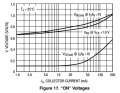# Transistor problem

#### FuneralHomeJanitor

Joined Oct 12, 2019
30
In the circuit given I have calculate the base-emitter and collector-emitter voltages for each transistor but there is no base resistance or collector resistance value given for the 3rd one from the left, how would I approach a problem like this when I don’t know these two values? There is nothing asking me to solve for Rb and Rc so is it something I am missing that involves the current gain? It is assumed the current gain is 200 in this problem. Thanks in advance.

#### MrChips

Joined Oct 2, 2009
22,553
The base-emitter junction needs to be forward biased for the transistor to conduct across the collector to emitter. Does that help you solve the problem?

(If the transistor is conducting you can assume that the base-emitter voltage is about 0.6V.)

#### FuneralHomeJanitor

Joined Oct 12, 2019
30
The base-emitter junction needs to be forward biased for the transistor to conduct across the collector to emitter. Does that help you solve the problem?

(If the transistor is conducting you can assume that the base-emitter voltage is about 0.6V.)
I just realized the base is grounded so it wouldn’t matter what the resistor values are if I am correct

#### ronsimpson

Joined Oct 7, 2019
1,125
Q1: There are many ways to do this. Here is one.
There is about 15V on Rb and about 15V on Rc. 100k & 10k so the Ic is 10x Ibase. The transistor is being used with a current gain of 10. Graph from the data sheet. Bottom line is Vce when the collector current is 10x the base current. Please read the Collector voltage from the graph. (voltage is the same for any current below 30mA)#### FuneralHomeJanitor

Joined Oct 12, 2019
30
This looks like home work so I will ask you for the answers.
Q2 is simple.
B-E voltage is ? You know this! (no base current)
Collector voltage is? (no base current so no collector current)
View attachment 219286
If you have Q2 right then Q3 is the same thing.
Collector voltage should be 15 V since it is not conducting in the cutoff region VCE = VCC? VBE should equal 0 V in this case if I understand correctly since there is no applied voltage at the base terminal in Q2 or Q3.